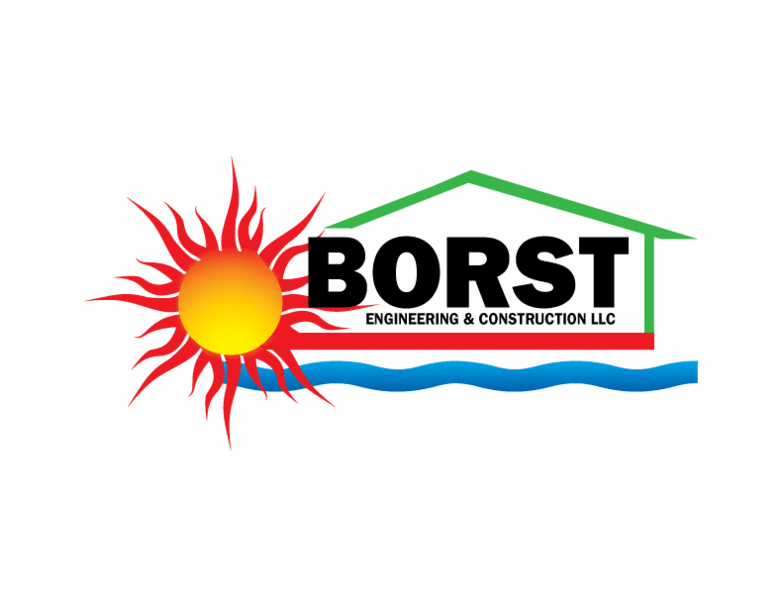Hire Borst Engineering for your ACCA Manual J or ASHRAE Heat Loss Analysis!
Heat Loss Analysis Calculator - Borst Engineering & Construction LLC
 Design Outdoor Dry Bulb Temp (Deg F) Design Indoor Dry Bulb Temp (Deg F) Window Area (SF) Window Total R-Value (Deg F-H-SF/BTU) Wall Area (SF) Wall Total R-Value (Deg F-H-SF/BTU) Door Area (SF) Door Total R-Value (Deg F-H-SF/BTU) Ceiling Area (SF) Ceiling Total R-Value (Deg F-H-SF/BTU) Floor Type (0=No Exposed Floor, 1=Framed Floor, 2=Basement Floor, 3=Slab-on-Grade Floor) Floor Area or Exposed Perimeter (SF or Feet) Floor Insulation R-Value (Deg F-H-SF/BTU) Building/Room Volume (CF or 0 for CFM) Infiltration Rate (ACH or CFM, 0.5 ACH typical for new construction)
 Window Heat Loss (BTU/Hour) Wall Heat Loss (BTU/Hour) Door Heat Loss (BTU/Hour) Ceiling Heat Loss (BTU/Hour) Floor Heat Loss (BTU/Hour) Infiltration Rate (CFM) Infiltration Heat Loss (BTU/Hour) Total Heat Loss (BTU/Hour) Total Heat Loss (BTU/Day) Total Heat Loss (BTU/Hour-Deg F)
Borst Engineering & Construction LLC